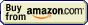# Titrations## Titration » Equivalence point calculation

Remember, that what we calculate is not an end point - but the equivalence point.

At the equivalence point calculations are based on the fact that there are stoichiometric amounts of titrant and titrated substance mixed in the solution. Thus for example in the case of acid-base titrations pH at the equivalence point is just a pH of salt solution (neutral for salt of strong acid and base, not neutral in the case of salts of weak acids or weak bases). Concentration of ions at the equivalence point of precipitation titration is identical with concentration of ions in equilibrium with the weakly soluble salt and is calculated from the solubility product - and so on.

Equivalence point calculations are discussed in more details in the appropriate sections:

Page was last modified on December 13 2009, 21:38:32.

titration at www.titrations.info © 2009 ChemBuddy